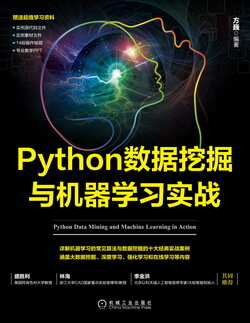# Python 数据挖掘与机器学习实战 (66)：回归分析介 3.5.2(数据预处理)

$HL\underline~PCT$（股票最高价与最低价变化百分比）：

$HL\underline~PCT=\frac{Adj.High-Adj.Close}{Adj.Close}~100.0\quad\quad\quad\quad\quad\quad\quad(3-9)$

$PCT\underline~change$（股票收盘价与开盘价的变化百分比）：

$PCT\underline~change=\frac{Adj.Close-Adj.Open}{Adj.Open}~100.0\quad\quad\quad\quad\quad\quad\quad(3-10)$

X = preprocessing.scale(X)

from sklearn.linear_model import LinearRegression

clf = LinearRegression(n_jobs=-1)

clf.fit(X_train, y_train)

forecast_set = clf.predict(X_lately)

accuracy = clf.score(X_test, y_test)